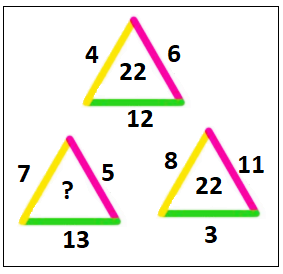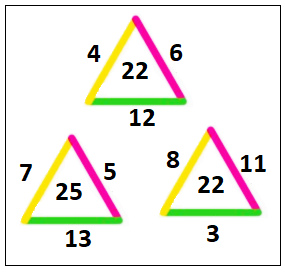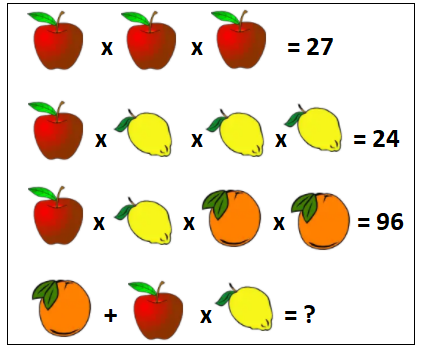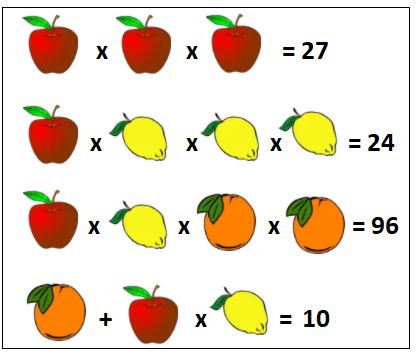Jet Set Go! All about Aeroplanes Jet Set Go! All about Aeroplanes

# Maths Puzzles

A maths puzzle is based on mathematical or numerical facts, rules, and objects, or whose solution requires strong mathematical reasoning, thought or consideration. Mathematics is the study of abstract topics such as numbers, shapes, quantity, space, and change. In simple words, it is the study of facts related to numbers and shapes; as there is no generally accepted definition for maths. There are a variety of views among mathematicians and scholars as to the specific scope and interpretation of mathematics.

Maths puzzles or Mathematical puzzles form an essential part of recreational mathematics. They have special rules, but they do not typically involve competition between two or more players. Preferably, the solver must find a solution that satisfies the given conditions to solve a particular puzzle. Maths puzzles require the basics of mathematics to solve them. A common type of math puzzle is logic puzzles, which are pretty much interesting for students not only in academics but also to work on in their leisure hours. Here, we are going to learn how to solve maths riddles in detail. Also, we have provided Maths riddles with answers to help students to solve the puzzles quickly.

## Maths Puzzles Tricks

There are some tricks and tips that help in solving different types of puzzles. The below steps will help you in solving any type of puzzles in maths.

Step 1: Take a quick look at the question.

Step 2: Analyse the statements and patterns given in the puzzle

Step 3: Develop a general idea regarding the theme of the problem.

Step 4: Select the data that is giving you some concrete or accurate information out of complete details given. Furthermore, select the data which helps in ruling out particular possibilities.

Step 5: Apply the possibilities to the given conditions of the puzzle

Step 6: The best possibility that satisfies the given conditions will be the solution to the puzzle

## Need of Solving Mathematical Puzzles

One may question the use of solving these puzzles. The answer is:

Solving math puzzles will help you to improve your mathematical skills. An interesting fact about maths puzzles is that the students who work with puzzles can perform better than those who do not, on tasks that evaluate their ability to revolve and transpose shapes. Maths puzzles are beneficial at every stage of students’ life either at the primary, secondary or higher secondary level.

At the primary and secondary level of education, puzzles are solved just for the sake of fun, though this develops a habit to apply our mind to every problem we encounter in our life. However, at the higher secondary level, this habit assists us in standing in the header row of the competition era of this world. Since we are already habituated to apply our mind, in this way, puzzles will help in enhancing our thinking skills instead of merely mugging up ability.

Below are a few examples of maths puzzles with answers. Maths riddles with answers will help you in understanding the variety of puzzles that involve logical and analytical skills to solve.

Example 1:

Solve the below Maths puzzle:Solution:

From the given, it can be observed that the addition of numbers exists.

In the top triangle, 4 + 6 + 12 = 22

In the second row, the second triangle has a sum of three numbers; 8 + 3 + 11 = 22

Thus, the missing number in the puzzle is:

7 + 5 + 13 = 25

Therefore,Example 2:

Solve the puzzle:Solution:

From the given, it can be observed that multiplication and addition are involved in the problem.

This can be solved by assuming some constant for each object and solving based on the condition given.

In the first row, each apple possesses the number 3 since 3 x 3 x 3 = 27

In the second row, let each mango hold “m” such that:

3 × m × m × m = 24

m3 = 24/3

m3 = 8

m = 2

Each mango = 2

In the third row:

Let each orange equal to “R”.

Thus, 3 × 2 × R × R = 96

6 × R2 = 96

R2 = 96/6

R2 = 16

R = 4

Each orange = 4

Finally, in the third row:

Orange + Apple × Mango = 4 + 3 × 2 = 4 + 6 = 10

Therefore,Stay tuned with BYJU’S- The Learning App and download the app to learn Maths-related concepts easily by exploring more videos.

## Frequently Asked Questions on Maths Puzzles

### What are Mathematical puzzles?

A puzzle is a thing that is difficult to comprehend or explain. A puzzle can be in the form of a game or toy to test creativity. Math puzzles are challenges that require logic and computation in order to solve.

### How do Maths riddles help children’s development?

Mathematical puzzles or Maths riddles for kids aid a child’s development in such a way that they are prepared not only for school exams, but also for life. The following are important aspects of a child’s development:
Skills in problem-solving
Ability to think analytically
Reasoning abilities
Connecting math to the real world
Using math in real-life circumstances

### Mention the different types of Maths Puzzles.

The different types of Math puzzles are number puzzles, crossword puzzles, logic puzzles, picture Puzzles, etc.

### What are the advantages of solving mathematical puzzles?

Children gain a number of benefits from solving arithmetic puzzles. Among these advantages are:
The kid begins to think logically, which allows them to consider many solutions to a problem.
By linking arithmetic to a real-life scenario, they become problem solvers.
Puzzle cards can assist the child in maintaining their level of interaction and developing their interest in mathematics

### What are number puzzles?

Number puzzles follow a set of rules, where one must first figure out the pattern and then answer the puzzle in accordance with the pattern.